## General Question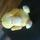# How does one find calculate pi?

Asked by RedDeerGuy1 (13895) February 7th, 2017

I was raised that it is 22 / 7. I was told that it is wrong. How do you find calculate pi beyond 3.14?

Observing members: 0Composing members: 0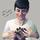Here’s one way that’s inefficient, but pretty simple:

1 – ⅓ + 1/5 – 1/7 + 1/9….

Continue that pattern into infinity and it will approach pi/4.

22/7 is a rational number that is kind of close to pi, but it is not equal to pi, which is an irrational number so cannot be expressed as a fraction.

Mariah (25831)“Great Answer” (9) Flag as…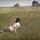Circumference divided by diameter. That’s the generic way to approximate pi. What @Mariah is describing is an infinite series, my favorite math concept.

ARE_you_kidding_me (17612)“Great Answer” (4) Flag as…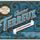Using the Monte Carlo method.

Basically, you inscribe a circle in a square with sides of arbitrary length. You know that the area of the square is the square of the length of its side. You assume that the area of the circle is some unknown constant times the square of its radius (the radius is half the length of the side of the square). This unknown constant is pi. The ratio of the area of the circle to the area of the square is then pi/4.

Let’s say that the square is centered on a Cartesian plane, so that its vertices are at (-0.5, 0.5), (0.5, 0.5), (0.5, -0.5), and (-0.5, -0.5). You can use a psuedo-random number generator to randomly plot points within that set of coordinates. A certain percentage of points will fall inside the perimeter of the circle. Given enough points, the percentage that falls inside of the circle will be proportional to the ratio of the area of the circle to the area of the square.

So this means that you can approximate pi by dividing the number of points that randomly fall within the circle by the total number of randomly generated points, and then multiplying that number by 4.

Here’s a video that explains it better.

cinnamonk (5367)“Great Answer” (5) Flag as…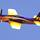@Mariah Has the most correct answer.

Rarebear (25154)“Great Answer” (1) Flag as…Response moderated (Spam)
Response moderated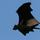We know that the relationship between circumference and radius is:
C = π x d
but let’s say that we don’t yet have a value for π.

If we wrap a string around a column, plate or other circular object of a known diameter, let’s say 1 meter, then we can mark or cut the length of string that makes one circumference at that diameter, and we have the value for π.

The measured value of the circumference, in this case where d = 1m, is π. The fractional approximation of 22 ÷ 7 is a fair value to use for most mortal purposes. As a carpenter (or even a backyard machinist) you’re not likely to need more than 3-place accuracy when measuring π.

CWOTUS (26067)“Great Answer” (3) Flag as…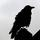^^That’s pretty much the way we used to do it when we made coffee tables out of wooden power company spools.

Espiritus_Corvus (17232)“Great Answer” (0) Flag as…Archimedes found an approximation to pi accurate to a few places. What he did was to inscribe polygons in a circle and compute the ratio of the perimeter of the polygon to the radius of the circle. As an upper bound, he used polygons that circumscribed the circle. There are formulas that give the sine and cosine of half of an angle x in terms of the sine and cosine of x. That allows you to start with a square or equilateral triangle and keep doubling the number of sides.

@Mariah‘s formula can be derived using calculus. See method 2 for this site

355/113 is a real nice approximation to pi accurate to six digits It is easy to memorize. Just write 113355 and split the number down the middle.

LostInParadise (25506)“Great Answer” (4) Flag as…or